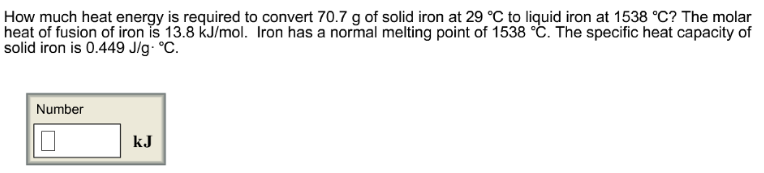# Problem: How much heat energy is required to convert 70.7 g of solid iron at 29 °C to liquid iron at 1538 °C? The molar heat of fusion of iron is 13.8 kJ/mol. Iron has a normal melting point of 1538 °C. The specific heat capacity of solid iron is 0.449 J/g °C.

###### FREE Expert Solution###### Problem Details

How much heat energy is required to convert 70.7 g of solid iron at 29 °C to liquid iron at 1538 °C? The molar heat of fusion of iron is 13.8 kJ/mol. Iron has a normal melting point of 1538 °C. The specific heat capacity of solid iron is 0.449 J/g °C.